# Eigenenergies of the Double-Well Potential¶

## Examples - Quantum Mechanics¶

Las edited: January 19th 2019

Potentials with two minima are often called double-well potentials. They have a wide range of applications in physics in general, including quantum mechanics. Two examples are:

• Hydrogen bonding: e.g. a proton (hydrogen ion) between the oxygen atoms of two water molecules will experience a double-well potential. Hence, it will tend to be closer to one of the two atoms.

• Inversion of ammonia: In ammonia, $NH_3$, the nitrogen atom is located above the plane made up by the three hydrogen atoms. The nitrogen atom can push through the plane of hydrogen, so that you now have an oppositely directed, but otherwise identical molecule. This could correspond to a specific double-well potential as shown in the figure above. You can read more about the inversion of ammonia in the Feynman Lectures on Physics online.The aim of this example is to calculate the eigenenergies of the lowest states for a one-dimensional double-well potential of the form

$$\qquad V(x) = \frac{V_\mathrm{max}}{b^4}(x^2-b^2)^2,$$

where $V_\mathrm{max}$ and $b$ are constants. This can be done with the method of forward shooting. We will use the same iterative formula as obtained in this example, which calculates the wave function at a point $x_{i+1}$ based on its value at the two previous points, according to

$$\qquad \psi_{i+1} = -\psi_{i-1} + 2\left[1 - (\Delta x)^2 \left(E-V(x_i)\right)\right] \psi_i,$$

where we have set $\hbar=m=1$ for simplicity. The first iteration has to be treated separately; using initial values $\psi_0$ and $\psi_0'$, $\psi_1$ can be calculated to lowest order from

$$\qquad \psi_1 = \psi_0 + \Delta x \cdot \psi_0'.$$

This then allows us to calculate $\psi_i$ for higher values of $i$. Since the potential is symmetric about $x=0$, we know that the wave functions must be either symmetric or anti-symmetric, with the $n$th excited state having parity $(-1)^n$ and $n$ nodes. This gives all the information we need in order to calculate the eigenenergies.

We will find the eigenenergies of the ground- and first excited state for two different values of $V_\mathrm{max}$ so as to see how the potential barrier affects the system. We choose the value $b=0.2$ throughout the whole example.

First, we import the modules needed. Then we define some functions which will be used to find the eigenenergies and to plot the wave functions:

• Results: a tuple used to return the results from the forward shooting.
• forwardShoot(): function which is used to calculate the eigenenergy and wave function for a given potential.
• normPlot(): normalizes and plots the wave function.

This spares us a lot of code writing since the same procedure is repeated for the ground- and first excited state for two different values of $V_\mathrm{max}$. Note that the function forwardShoot() only works for the ground- and first excited state as it is defined now. (In fact, it works for all states where the wave function $\psi_n$ approaches 0 from above for large values of $x$, i.e. $\lim_{x \to \infty} \psi_n(x) \rightarrow 0^{+}$. This is the case for $\psi_n$ with $n \in \{0,1,4,5,8,9,\dots\}$.) To work for the second and third excited states, the conditions used for the bisection method would have to be changed.

In :
%matplotlib inline
import numpy as np
import matplotlib.pyplot as plt
from collections import namedtuple

# Set common figure parameters:
newparams = {'axes.labelsize': 14, 'axes.linewidth': 1, 'savefig.dpi': 300,
'lines.linewidth': 1.0, 'figure.figsize': (8, 3),
'figure.subplot.wspace': 0.4, 'figure.dpi': 200,
'ytick.labelsize': 10, 'xtick.labelsize': 10,
'legend.fontsize': 10, 'legend.frameon': False,
'legend.handlelength': 1.5}
plt.rcParams.update(newparams)

In :
Results = namedtuple('res', ['func','E'])

def forwardShoot(V, dx, psi_0, dpsi_0, E_l=0.0, E_u=400.0, acc=1e-10):
"""Takes a potential and a vector of zeroes and uses the forward shooting method to calculate the eigenenergy
using the upper and lower bounds given.

Arguments:
V       Potential
dx      Step length x-axis
psi_0   Initial value of wavefunction at x=0
dpsi_0  Initial value of first derivative of wavefunction at x=0
E_l     Lower bound of energy
E_u     Upper bound of energy
acc     Accuracy when determining the energy

Returns tuple with elements
func    Wavefunction
E       Eigenenergy
"""

f = np.zeros(len(V))
f = psi_0
df_0 = dpsi_0

E1 = E_l  # Lower bound for eigenenergy
E2 = E_u  # Upper bound for eigenenergy
E = E1
DeltaE = 1
while DeltaE > acc:
for i, V_ in enumerate(V[0:-1]):
if i==0:
f[i+1]=f[i]+dx*df_0
else:
f[i+1] = -f[i-1]+2*f[i]*(1-dx**2*(E-V_))
# Implementation of bisection method. If the function value is out of bounds,
# a new value for the energy is chosen. When the difference between the upper
# and lower bound for the energy is smaller than the given accuracy,
# the while loop stops, yielding a result for E.
if f[i] > 20:
E1 = E
E = E1 + (E2-E1)/2
break
elif f[i] <- 20:
E2 = E
E = E2 - (E2-E1)/2
break
DeltaE = E2-E1
res = Results(f, E)
return res

def normPlot(V, func, E, x, x_end, title='', sym=True, legend_loc=1):
"""Normalizes and plots the wavefunction together with the potential and eigenenergy.

Arguments:
V            Potential
func         Wavefunction
E            Eigenenergy
x            Vector with x-values
x_end        Biggest x-value to be included in normalization/plot
title        Plot title
sym          True if symmetric wavefunction, false if anti-symmetric
legend_loc   Placement of plot legend

Returns:
F            Normalized wavefunction.
"""

dx = x-x
sign = -1
if sym: sign = 1
c = sum(2*func[0:int(x_end/dx)]**2*dx)
F = func/np.sqrt(c)

plt.figure()
p1, = plt.plot(x, V/V, 'b')
p2, = plt.plot(x, F, 'g')
p3, = plt.plot([-x_end,x_end], [E/V, E/V], 'r--')
plt.legend([p1,p2,p3], [r'Potential $V(x)/V_\mathrm{max}$',r'Wave function $\psi(x)$',
r'Eigenenergy $E/V_\mathrm{max}$'], loc=legend_loc)
plt.plot(-x, V/V, 'b', -x, sign*F, 'g', [-x_end,x_end], [0,0], 'k:')
plt.ylim([min(sign*F[0:int(x_end/dx)])-0.1, max(F[0:int(x_end/dx)])+0.5])
plt.xlim([-x_end, x_end])
plt.xlabel(r'$x$')
plt.title(title)

return F


### Potential barrier $V_\mathrm{max} = 40$¶

#### Ground State¶

With $n=0$, the ground state has even parity and is hence symmetric about the origin. This means that the initial condition for $\psi'$ is $\psi_0' = 0$, while we can choose $\psi_0$ freely, e.g. $\psi_0 = 1$, since this is only a matter of normalization. The energy is calculated, using the bisection method, with lower bound $E=0$, and upper bound $E=40$, since it is reasonable to assume that there exists a state with energy $E<V_\mathrm{max}$. This leads to the following code, where the previously defined functions are used:

In :
b = 0.2
Vmax = 40

n = 1000                     # Number of points per unit on x-axis
dx = 1.0/n                   # Step length
p = 1.0                      # Which x-values to include
x = np.linspace(0, p, p*n, True)

V = Vmax/b**4*(x**2-b**2)**2 # Potential V(x)

f_0 = 1.0                    # Initial value psi_0
df_0 = 0.0                   # Initial value psi'_0

res1 = forwardShoot(V=V, dx=dx, psi_0=f_0, dpsi_0=df_0, E_u=40)
E1_0 = res1.E
print(r'The energy is: %s' % E1_0)

The energy is: 29.920959198534547

/Library/Frameworks/Python.framework/Versions/3.5/lib/python3.5/site-packages/ipykernel_launcher.py:7: DeprecationWarning: object of type <class 'float'> cannot be safely interpreted as an integer.
import sys


The resulting energy is $E_0=29.92$. We now normalize and plot the wave function. When normalizing, we want to avoid the inclusion of domains where the wave function blows up. In other words, we want to include only values of $x$ where $\psi$ is well-behaved. In this case, we find by inspection that $x \in [-0.7, 0.7]$.

In :
F0 = normPlot(V=V, func=res1.func, E=E1_0, x=x, x_end=0.7, title=r'Ground state, $V_\mathrm{max}=40$')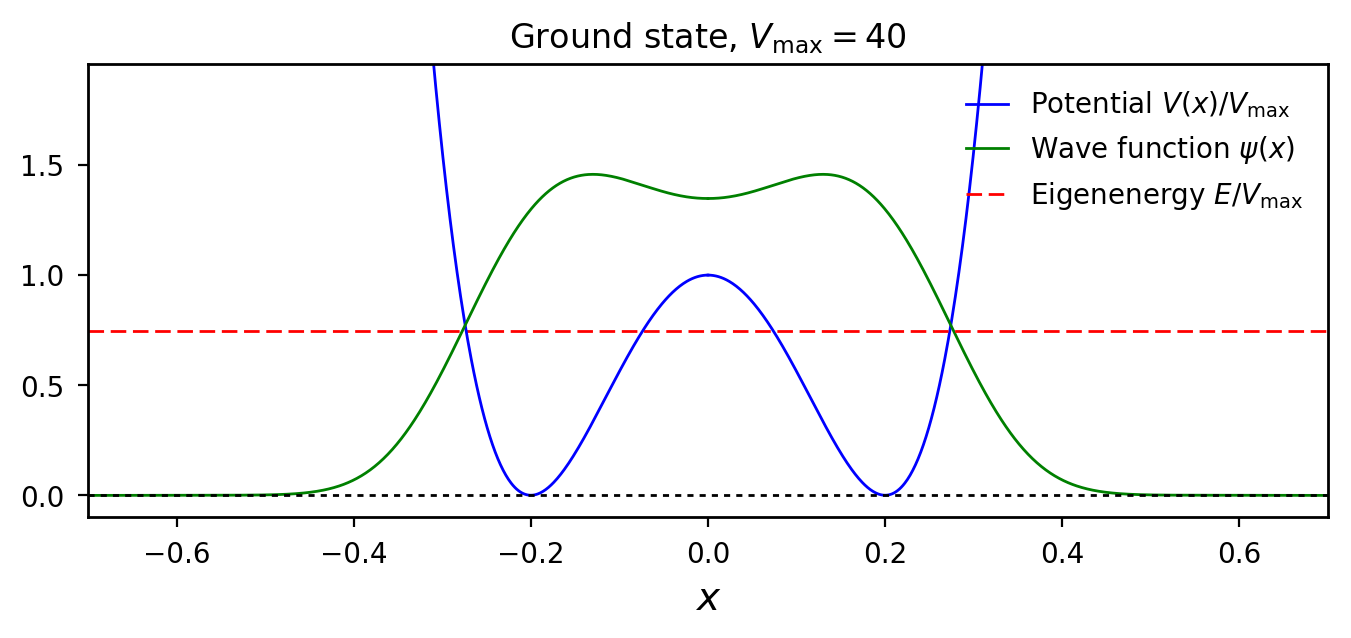#### First excited state¶

Due to symmetry arguments, we must now have $\psi_0 = 0$, while we can choose $\psi_0'$ freely, e.g. $\psi_0' = 4$ as in the code below. Here, the lower bound for the energy is the ground state energy, while we choose the upper bound to be $100$.

In :
# Initial values:
f_0 = 0.0
df_0 = 1

res2 = forwardShoot(V=V, dx=dx, psi_0=f_0, dpsi_0=df_0, E_l=E1_0, E_u=100)
E1_1 = res2.E
print('The energy is: %s' % E1_1)

The energy is: 48.4381461985486


The energy of the first excited state is $E_1=48.44$. Again we normalize and plot the wavefunction.

In :
F1 = normPlot(V=V, func=res2.func, E=E1_1, x=x, x_end=0.6, title=r'First excited state, $V_\mathrm{max}=40$',
sym=False, legend_loc=4)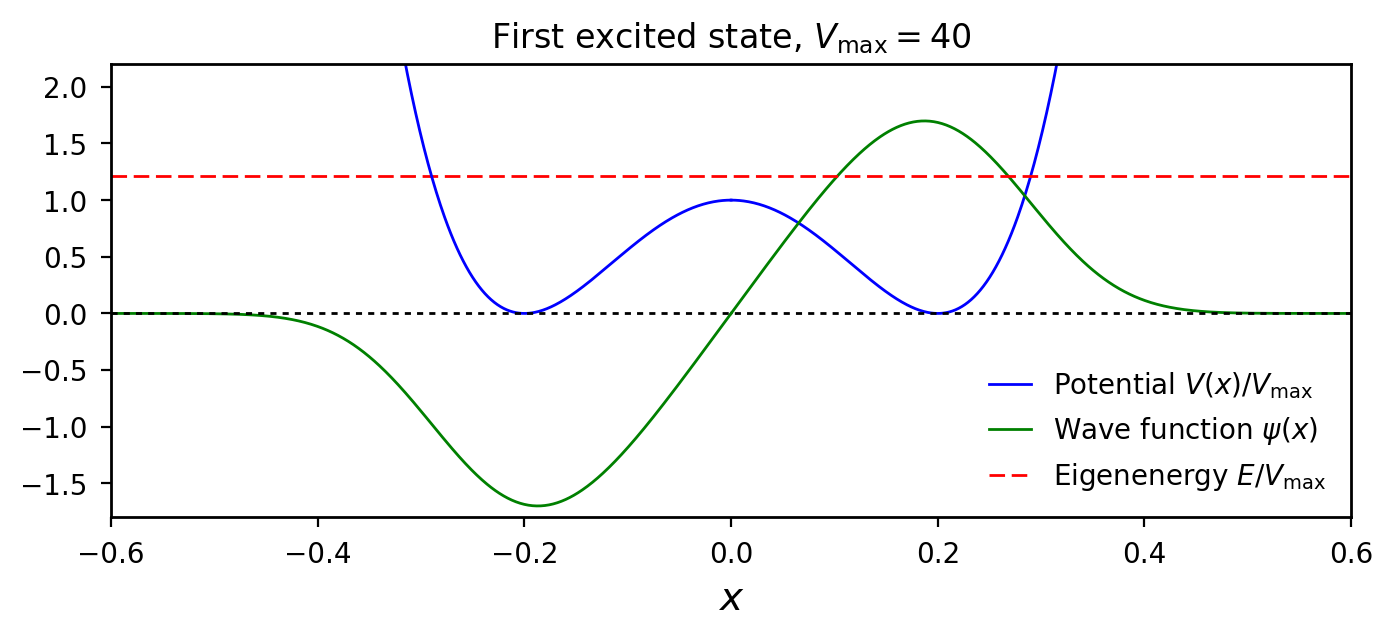As we see there is a large difference between the eigenenergies and wave functions of the two (ground and first excited) states. Let us now increase the potential barrier and see how this affects the system.

### Potential barrier $V_\mathrm{max} = 400$¶

#### Ground state¶

Since the potential barrier is much higher in this case, leading to a wave function of increased curvature, we will choose a smaller initial value $\psi_0=0.1$, while $\psi_0' = 0$ as before. Once again, we choose $V_\mathrm{max}$ as an upper bound for the energy.

In :
Vmax = 400
V = Vmax/b**4*(x**2-b**2)**2

# Initial values
g_0 = 0.1
dg_0 = 0.0

res3 = forwardShoot(V=V, dx=dx, psi_0=g_0, dpsi_0=dg_0, E_u=400)
E2_0 = res3.E

print('The energy is: %s' % E2_0)

The energy is: 133.96371872663622


The resulting energy is $E_0 = 133.96$. Plotting the normalized wavefunction:

In :
G0 = normPlot(V=V, func=res3.func, E=E2_0, x=x, x_end=0.5, title=r'Ground state, $V_\mathrm{max}=400$', legend_loc=9)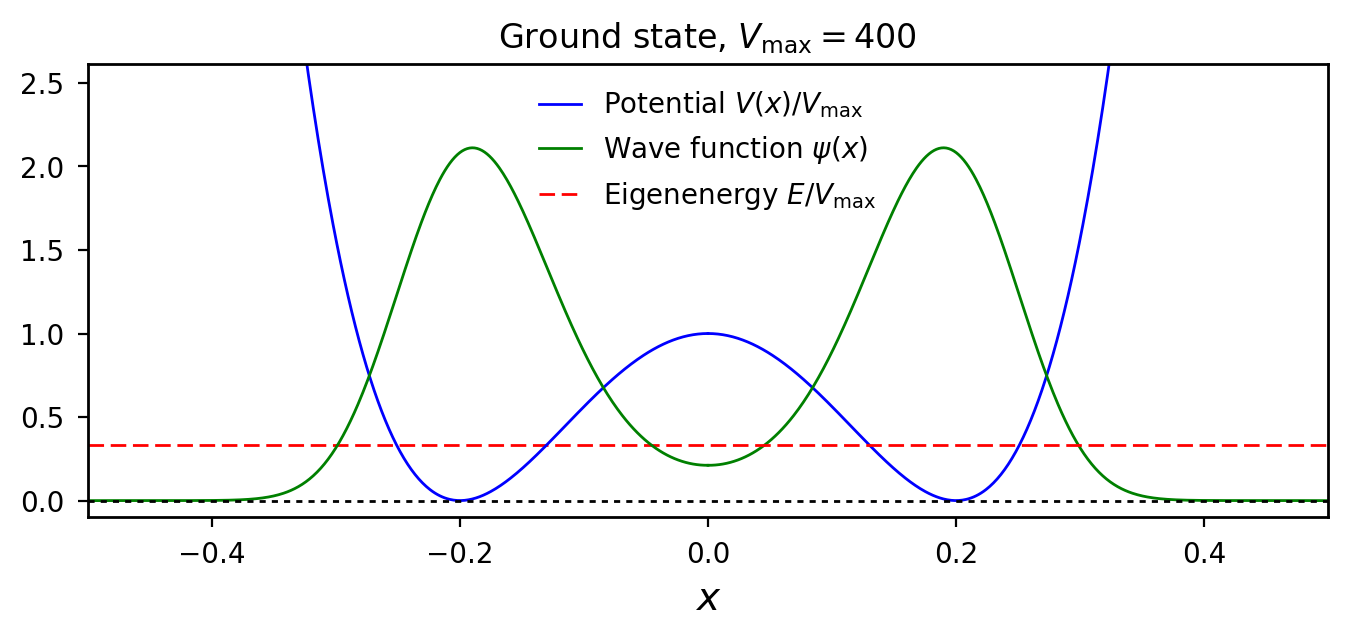#### First excited state¶

In :
# Initial conditions
g_0 = 0.0
dg_0 = 1

res4 = forwardShoot(V=V, dx=dx, psi_0=g_0, dpsi_0=dg_0, E_l=E2_0, E_u=400)
E2_1 = res4.E

print('The energy is: %s ' % E2_1)

The energy is: 134.95475614467142


The resulting energy is $E_0 = 134.95$ and now only slightly higher than the ground state energy. Plotting the normalized wavefunction:

In :
G1 = normPlot(V=V, func=res4.func, E=E2_1, x=x, x_end=0.5, title=r'First excited state, $V_\mathrm{max}=400$',
sym=False, legend_loc=4)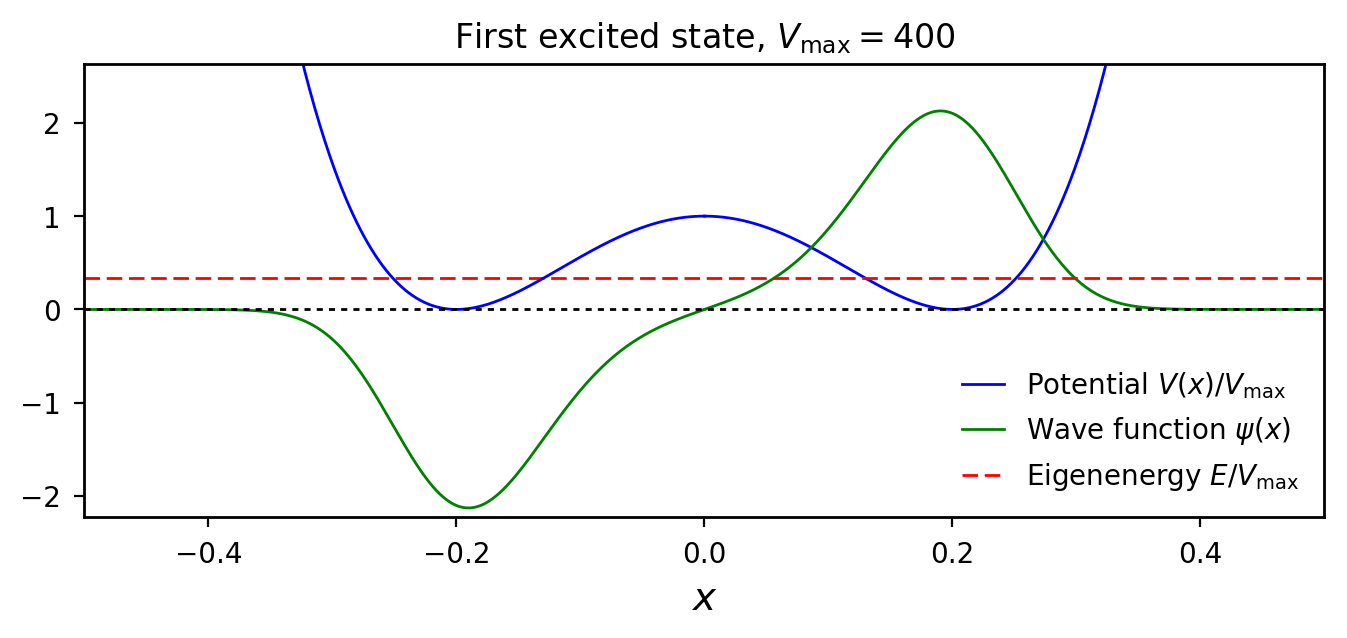We have now determined four energies and wave functions. Let us plot the two wave functions which correspond to the same potential barrier, together in the same plot so as to simplify comparisons.

In :
plt.figure()
p1, = plt.plot(x, F0, 'b')
p2, = plt.plot(x, F1, 'r')
plt.legend([p1,p2], ['Ground State','First Excited State'])
plt.plot(-x, F0, 'b', -x, -F1, 'r', [-0.5,0.5], [0,0], 'g:')
plt.ylabel(r'$\psi(x)$')
plt.xlabel(r'$x$')
plt.ylim([-2.0, 2.5])
plt.xlim([-0.5, 0.5])
plt.title(r'Wavefunctions for $V_\mathrm{max}=40$')

plt.figure()
p1, = plt.plot(x, G0, 'b')
p2, = plt.plot(x, G1, 'r')
plt.legend([p1,p2], [r'Ground State','First Excited State'])
plt.plot(-x, G0, 'b', -x, -G1, 'r', [-0.5,0.5], [0,0], 'g:')
plt.ylabel(r'$\psi(x)$')
plt.xlabel(r'$x$')
plt.ylim([-2.5, 3.2])
plt.xlim([-0.5, 0.5])
plt.title(r'Wavefunctions for $V_{max}=400$');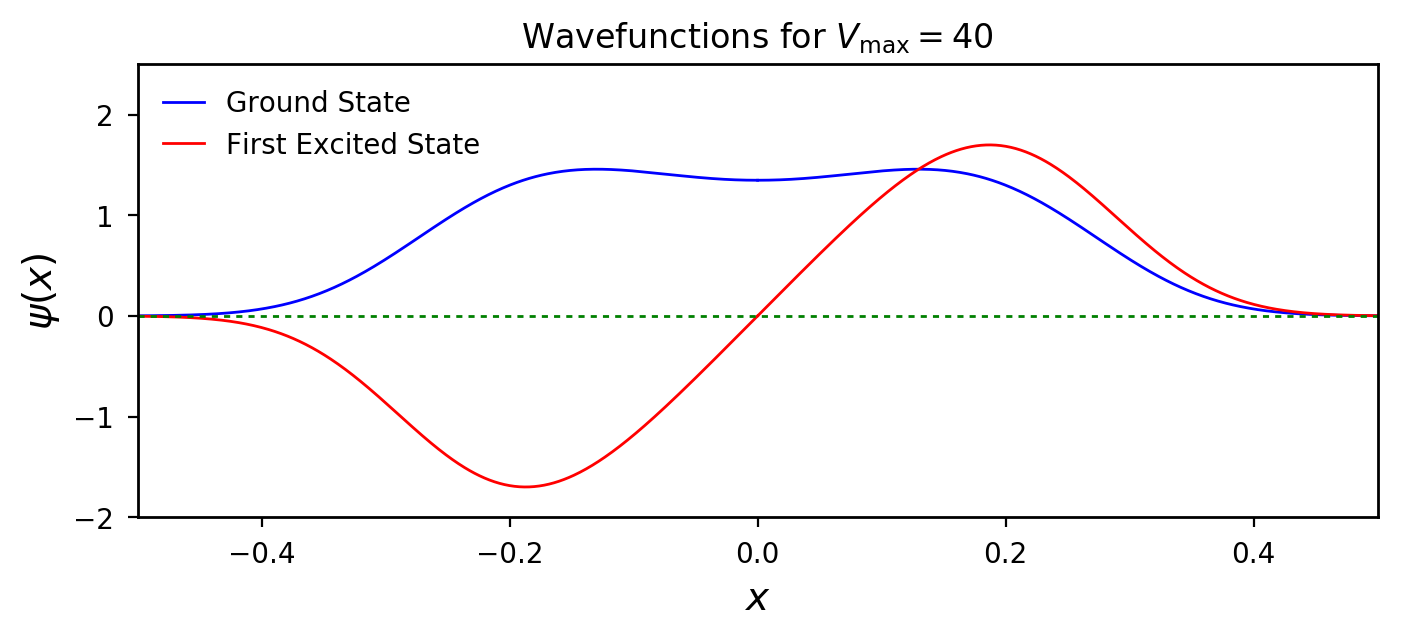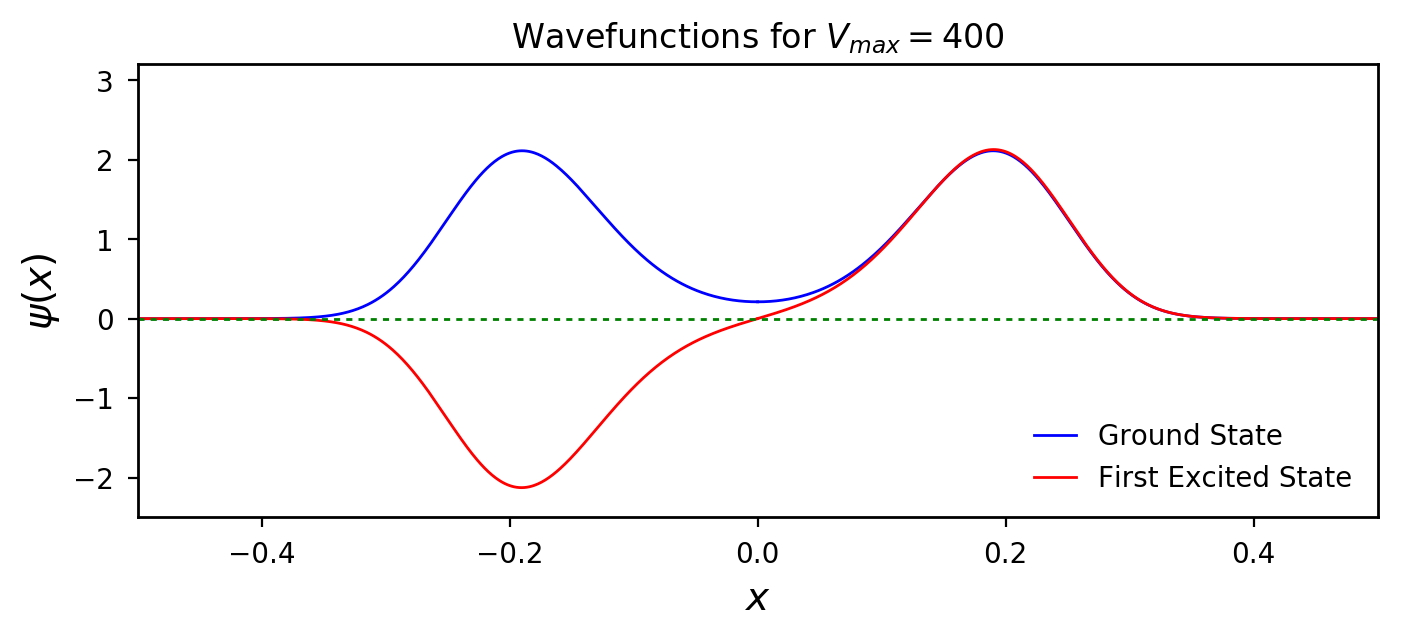We see that the difference between the wave functions for $V_\mathrm{max}=40$ is large and we have $E_0/E_1 \approx 0.6$. For $V_{max}=400$ on the other hand, we see that the wave functions are almost identical for $x>0$ and if we plot the absolute square of these two functions, we obtain almost the exact same function, as seen from the plot below. We also have $E_0/E_1 \approx 1$.

In :
plt.figure()
pp1, = plt.plot(x, G0**2, 'b')
p2, = plt.plot(x, G1**2, 'r')
plt.legend([p1,p2], ['Ground State','First Excited State'])
plt.plot(-x, G0**2, 'b', -x, G1**2, 'r', [-0.5,0.5], [0,0], 'g:')
plt.ylabel(r'$\psi(x)$')
plt.xlabel(r'$x$')
plt.ylim([-0.1, 5.5])
plt.xlim([-0.5, 0.5])
plt.title(r'Absolute Square of Wavefunctions for $V_\mathrm{max}=400$');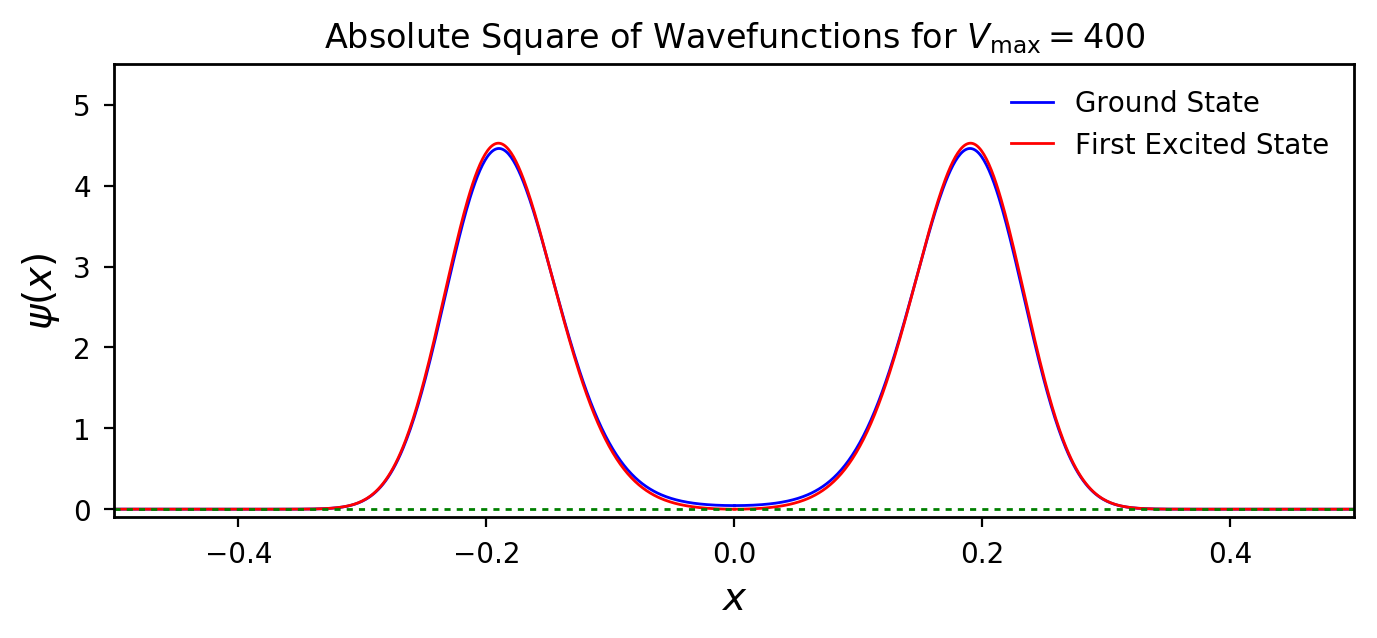We also see that with the higher potential barrier, the probability of the particle being in the classically forbidden region, is dramatically reduced for the ground state, as it should be. Also note that since the energies and the absolute squares of the two wave functions are so similar when $V_\mathrm{max} = 400$, it is possible to construct wave functions such that the particle is almost certainly either in the left or the right well, given by $\psi_0 - \psi_1$ and $\psi_0 + \psi_1$ respectively, as seen in the following plot.

In :
plt.figure()
p1, = plt.plot(x, 0.5*(G0-G1)**2,'b')
p2, = plt.plot(x, 0.5*(G0+G1)**2,'r')
plt.legend([p1,p2], [r'$|\psi_0 - \psi_1|^2/2$',r'$|\psi_0 + \psi_1|^2/2$'])
plt.plot(-x, 0.5*(G0+G1)**2,'b', -x, 0.5*(G0-G1)**2, 'r', [-0.5,0.5], [0,0], 'g:')
plt.ylabel(r'$\psi(x)$')
plt.xlabel(r'$x$')
plt.ylim([-0.1, 10.0])
plt.xlim([-0.5, 0.5]);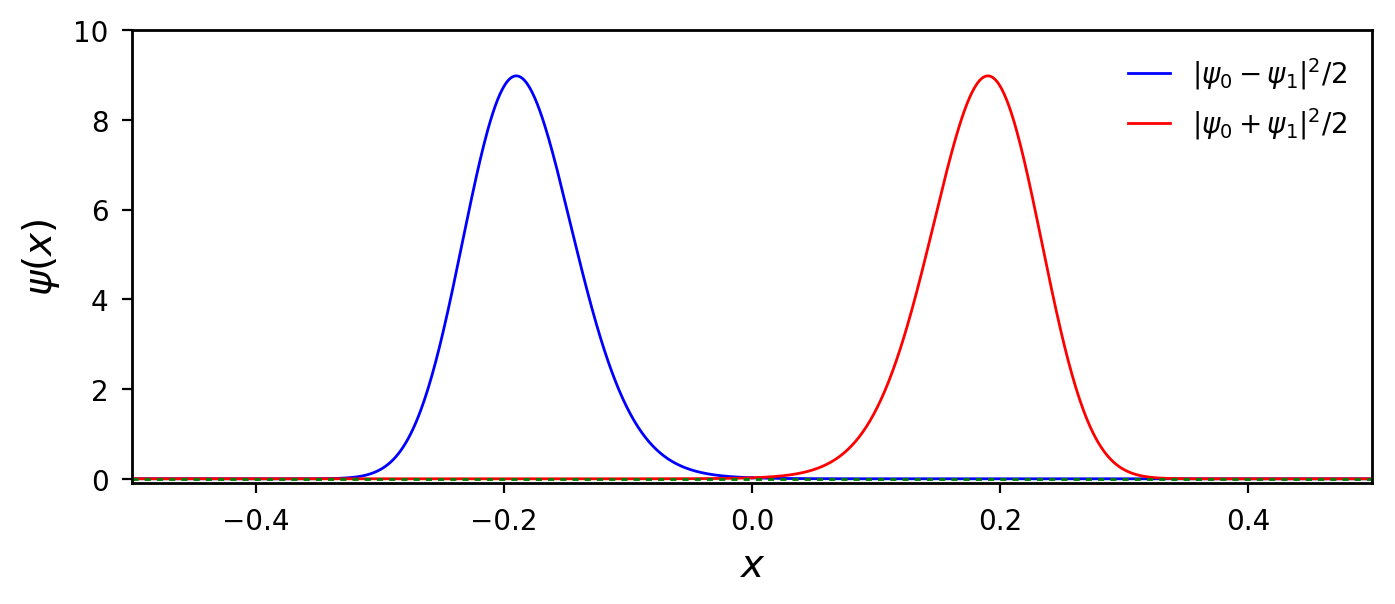In other words, as the potential barrier increases, the situation resembles more and more the situation of a particle being confined in one of two separate wells.

One might wonder: how large is the energy of the second excited state for $V_\mathrm{max}=400$? Is it close to $E_0$ and $E_1$? To find out, we need to make a small change to the function forwardShoot():

In :
def forwardShoot2(V, dx, psi_0, dpsi_0, E_l=0.0, E_u=400.0, acc=1e-10):
"""Takes a potential and a vector of zeroes and uses the forward shooting method to calculate the eigenenergy
using the upper and lower bounds given.

Arguments:
V       Potential
dx      Step length x-axis
psi_0   Initial value of wavefunction at x=0
dpsi_0  Initial value of first derivative of wavefunction at x=0
E_l     Lower bound of energy
E_u     Upper bound of energy
acc     Accuracy when determining the energy

Returns tuple with elements
func    Wavefunction
E       Eigenenergy
"""

f = np.zeros(len(V))
f = psi_0
df_0 = dpsi_0

E1 = E_l  # Lower bound for eigenenergy
E2 = E_u  # Upper bound for eigenenergy
E = E1
DeltaE = 1
while DeltaE > acc:
for i, V_ in enumerate(V[0:-1]):
if i==0:
f[i+1]=f[i]+dx*df_0
else:
f[i+1] = -f[i-1]+2*f[i]*(1-dx**2*(E-V_))
# Implementation of bisection method. If the function value is out of bounds,
# a new value for the energy is chosen. When the difference between the upper
# and lower bound for the energy is smaller than the given accuracy,
# the while loop stops, yielding a result for E.
if f[i] > 20:
# Changes here:
E2 = E
E = E2 - (E2-E1)/2
break
elif f[i] <- 20:
# Changes here:
E1 = E
E = E1 + (E2-E1)/2
break
DeltaE = E2-E1
res = Results(f, E)
return res


We know that the second excited state should be symmetric about the origin. Hence, we can use the same initial conditions as for the ground state. We also use the energy of the first excited state as a lower bound.

In :
g_0 = 0.1
dg_0 = 0

res5 = forwardShoot2(V=V, dx=dx, psi_0=g_0, dpsi_0=dg_0, E_l=E2_1, E_u=400)
E2_2 = res5.E
print(r'The energy is: %s' % E2_2)

The energy is: 349.0778483719083


The energy is $E_2=349.08$, a big increase compared to $E_1$!

In :
G2 = normPlot(V, res5.func, E2_2, x, 0.5, title=r'Second excited state, $V_\mathrm{max}=400$', sym=True, legend_loc=8)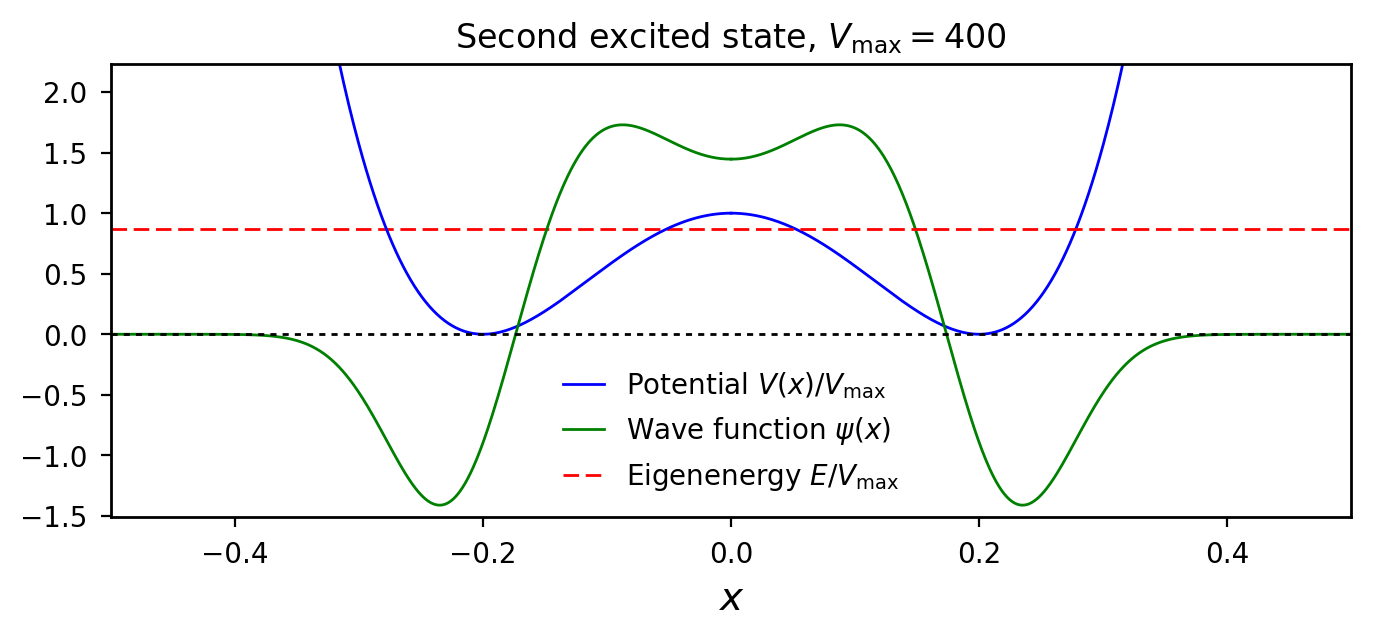We now see that the wave function has changed dramatically from that of the two lower states. Near the origin, it is now more similar to that of the ground state for $V_\mathrm{max}=40$. This seems reasonable considering that the energy is now comparable to the potential barrier, which was also the case for the ground state with lower potential barrier. A particle is no longer confined to the two wells since there is now a considerable probability of the particle being between the wells, meaning in the classically forbidden area.